You can find my final code here and video results here

Problem

Most state of the art image classifiers uses some form of Convolutional Neural Network (CNN). For these applications, there are specific labels that the neural network is trying to classify (i.e classifying cat and dogs). To achieve this, a fully connected layer must be included at the end of the network. Usually, this layer is a softmax layer which gives you the probability of each label. However, this fully connected layer removes all spatial information. There is no way to determine where the object is located in the image.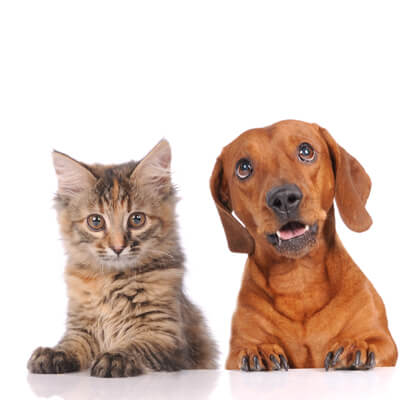Also, CNN cannot detect multiple objects in an image. One method to solve both of these problems is to use the sliding window method which is to split the image into small subset images and run these new small images through the CNN which I describe here in another posts. However, this is extremely computational expensive since one image can become hundreds and create results that are sub-optimal since the size and location of the subset images can greatly alter the results. As the demand for “smarter” image classifier grows, there are new cutting edge architectures that can classify images while preserving spatial information.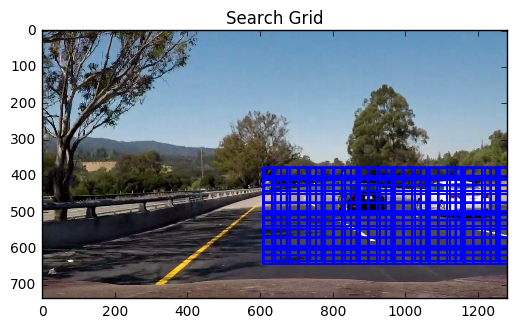Solution

There are many possible solutions to this problem such as one look algorithms (like YOLO, which I address in another posts), Mask R-CNN, and Semantic Segmentation. For this posts, I will be explaining Fully Connected Neural Network and how to apply it to Semantic Segmentation.

Overall Idea

The main idea of semantic segmentation is to create a neural network where you input any image and it outputs an image mask. An image mask is essentially a “colored pencil” version of the image, where each object is specifically colored to a certain color to identify the location and label.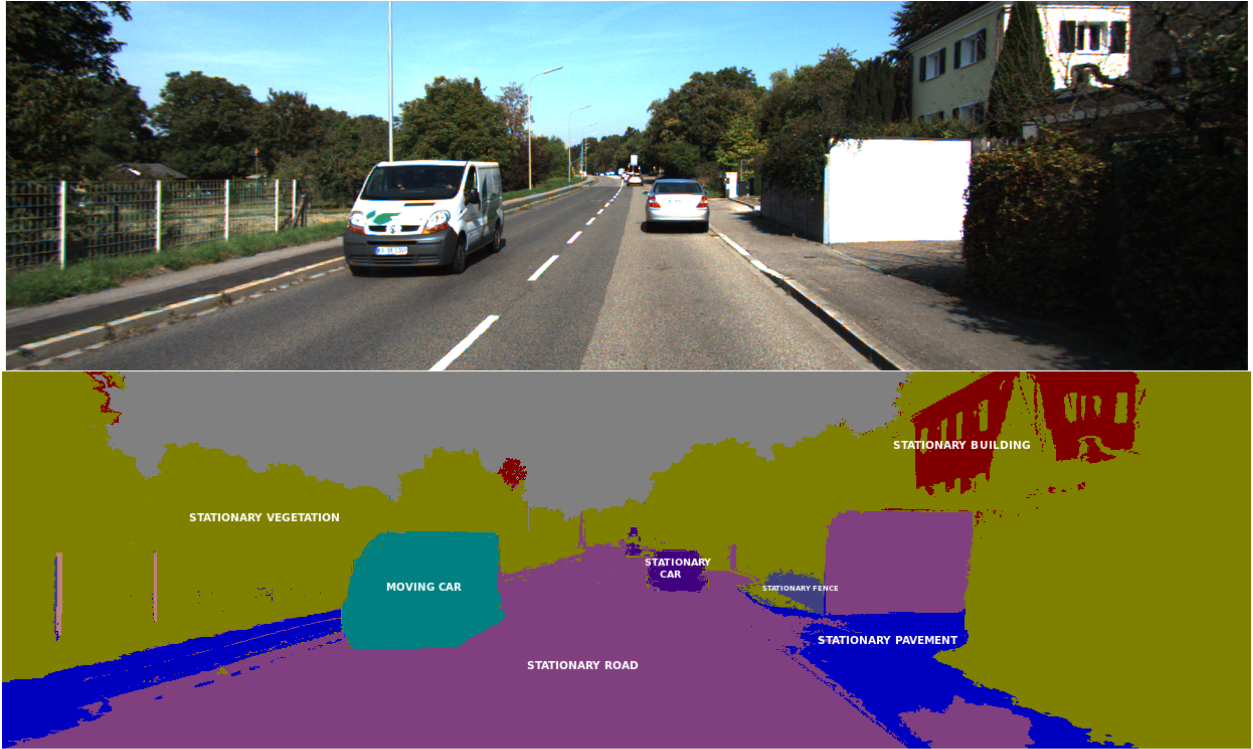Source

Fully Convolutional Network (FCN)

A FCN is essentially an encoder and decoder stacked together. However, unlike a traditional encoder/decoder where the image input and output are the same, a FCN encodes your input image and decodes the input into an image mask as mentioned before. The encoder section is just a regular CNN without the last fully connected layer. Since the encoder is like a regular CNN, the input to the encoder is an image (usually in RGB colorspace). The decoder section is called a deconvolutional neural network (DCNN). As the name implies, its the reverse of a CNN. Instead of downsampling the image through a series of transpose kernels and pooling, the DCNN upsamples the image through a series of kernels and “unpooling”.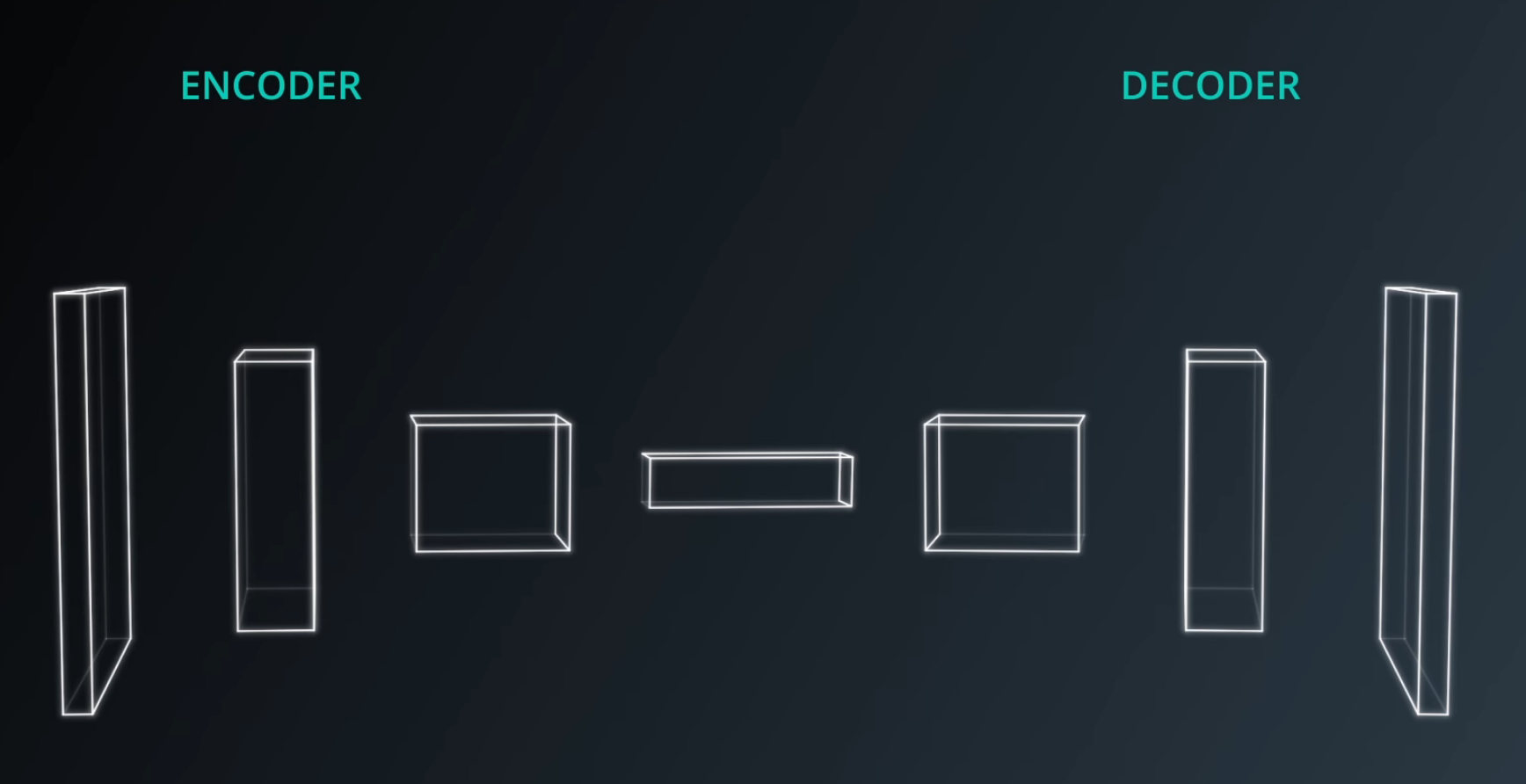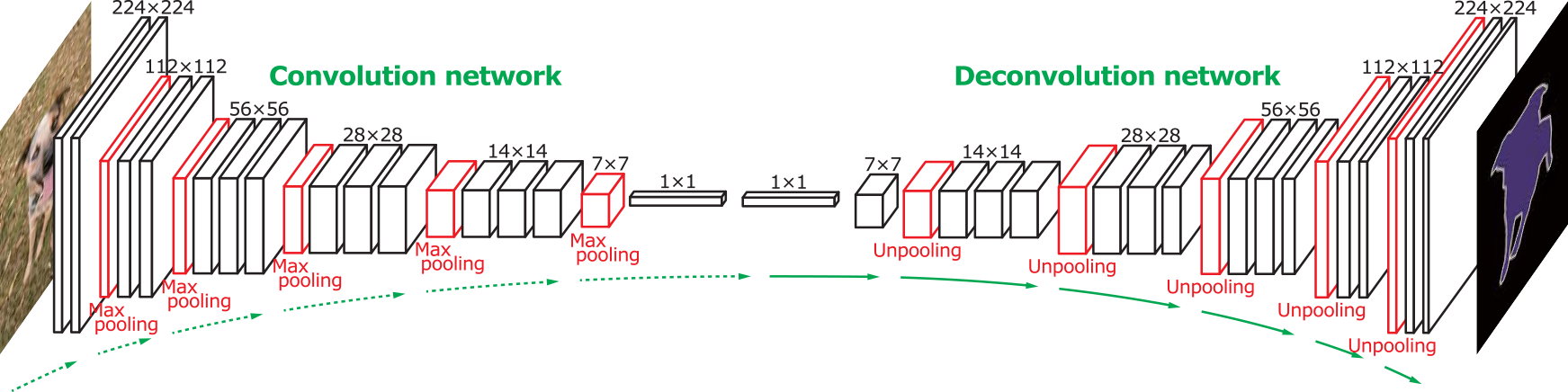Source

There are many limitations using the network as described before. First, the final mask results tend to be bad, where the lines around the object is not clear. Also, the model tends to generalize poorly since some of the complex spatial feature can be lost due to the pooling. In addition, the current network structure can only be trained with the same sized image and mask.

To solve all these problems, skipping layers are introduced. Skipping layers provide different features from the encoder part into the decoder part so that the decoder can learn high level spatial features that might be lost throughout the process otherwise. Another benefit from these skip connections is that this network can predict and train with any image size (though it is usually recommended to train with consistent image sizes).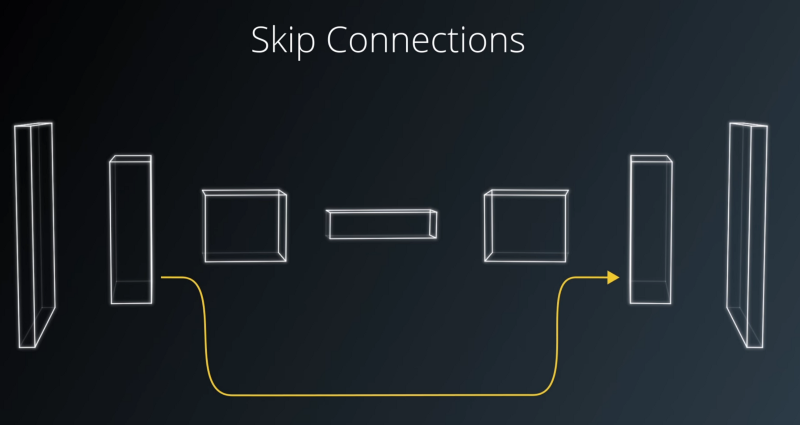Training FCN

Semantic segmentation are rarely trained from scratch since it takes a long time to achieve acceptable results. One popular method is to use a pre-trained model for the encoder since there are many popular pre-trained CNN available on the internet that perform great for classifications (>99% accuracy). Therefore, you will only need to train the decoder portion of the network. The final results will be better than training from scratch and training time is substantially shorter.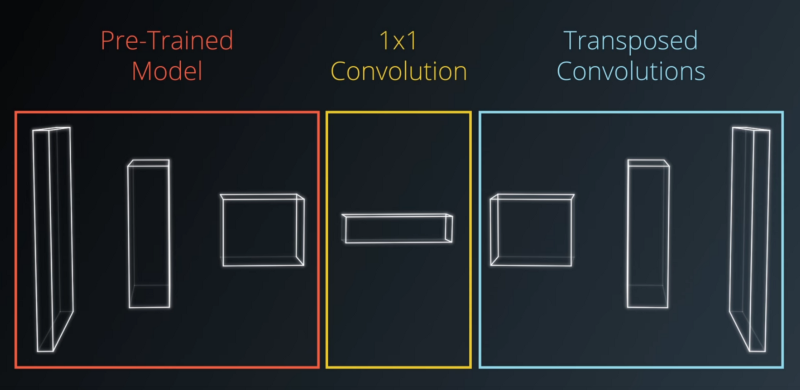Overall Procedure

These are the following steps to train your own custom semantic segmentation model using the method I proposed. It it usually easier to use a “lower” level library like Tensorflow and PyTorch rather than a higher level library like Keras when re-purposing certain architectures in part of your network.

1) Choose a popular pre-trained model (GoogLeNet, AlexNet, VGG16, etc) and load the model architecture and weights. 2) Split up the layers in the model architecture and name them so that you can call them easily 3) Remove the last fully connected layer from the network and add a 1x1 convolution network 4) Add the decoder section into the model 5) Add all the required skipping layers.

Note, the last two steps is not arbitrary. Similar to how CNN have specific architectures, it is usually wise to follow a research paper to get good results. However, you can play with the network to fit your needs.

FCN-8

For this part, we will be following UC Berkeley’s FCN-8 architecture and using the VGG16 pre-trained network. You can read more about this on their paper

To use the pretrained CNN, it is vital to keep track of the name and layers you will need since the DCNN will require it for the skipping layers. Also, the weights for those layers will need to be loaded since we will be fine tuning their values. Luckily, Tensorflow (and PyTorch) has preloaded model architecture so loading the model is quick and easy. For FCN-8, we will be loading layer 1 (image input layer), 3, 4, and 7.

 1 2 3 4 5 6 7 8 9 10 11 12 13 14 15 16 17 18 19 20 21 22 23 24 25 26 27 28 29 30 31 32 33 34 35 36 37 38 39 40 41 42 43 44 45 def load_vgg(sess, vgg_path): """ Load Pretrained VGG Model into TensorFlow. :param sess: TensorFlow Session :param vgg_path: Path to vgg folder, containing "variables/" and "saved_model.pb" :return: Tuple of Tensors from VGG model (image_input, keep_prob, layer3_out, layer4_out, layer7_out) """ # TODO: Implement function # Use tf.saved_model.loader.load to load the model and weights vgg_tag = 'vgg16' vgg_input_tensor_name = 'image_input:0' vgg_keep_prob_tensor_name = 'keep_prob:0' vgg_layer3_out_tensor_name = 'layer3_out:0' vgg_layer4_out_tensor_name = 'layer4_out:0' vgg_layer7_out_tensor_name = 'layer7_out:0' tf.saved_model.loader.load(sess, [vgg_tag],vgg_path) graph = tf.get_default_graph() w1 = graph.get_tensor_by_name(vgg_input_tensor_name) keep = graph.get_tensor_by_name(vgg_keep_prob_tensor_name) w3 = graph.get_tensor_by_name(vgg_layer3_out_tensor_name) w4 = graph.get_tensor_by_name(vgg_layer4_out_tensor_name) w7 = graph.get_tensor_by_name(vgg_layer7_out_tensor_name) return w1, keep, w3, w4, w7

Creating the 1x1 Convolutional Layer with Layer Skipping

For the FCN-8, there is no up-scaling until the final layer to match the mask image size. The main innovation of the FCN-8 is the three consecutive 1x1 layers added at the end of VGG16 network. Each of the consecutive layers are connected in series with layer skipping from layer 7, 4, and 3 respectively.

 1 2 3 4 5 6 7 8 9 10 11 12 13 14 15 16 17 18 19 20 21 22 23 24 25 26 27 28 29 30 31 32 33 34 35 36 37 38 39 40 41 42 def layers(vgg_layer3_out, vgg_layer4_out, vgg_layer7_out, num_classes): """ Create the layers for a fully convolutional network. Build skip-layers using the vgg layers. :param vgg_layer3_out: TF Tensor for VGG Layer 3 output :param vgg_layer4_out: TF Tensor for VGG Layer 4 output :param vgg_layer7_out: TF Tensor for VGG Layer 7 output :param num_classes: Number of classes to classify :return: The Tensor for the last layer of output """ # TODO: Implement function #Encoder with FCN conv_1x1_7 = tf.layers.conv2d(vgg_layer7_out, num_classes, 1, padding='same',kernel_regularizer=tf.contrib.layers.l2_regularizer(1e-3)) conv_1x1_4 = tf.layers.conv2d(vgg_layer4_out, num_classes, 1, padding='same',kernel_regularizer=tf.contrib.layers.l2_regularizer(1e-3)) conv_1x1_3 = tf.layers.conv2d(vgg_layer3_out, num_classes, 1, padding='same',kernel_regularizer=tf.contrib.layers.l2_regularizer(1e-3)) #Decoder output = tf.layers.conv2d_transpose(conv_1x1_7,num_classes, 4, 2, padding='same', kernel_regularizer=tf.contrib.layers.l2_regularizer(1e-3)) input = tf.add(output, conv_1x1_4) input = tf.layers.conv2d_transpose(input, num_classes, 4, strides=(2, 2), padding='same', kernel_regularizer=tf.contrib.layers.l2_regularizer(1e-3)) input = tf.add(input, conv_1x1_3) Input = tf.layers.conv2d_transpose(input, num_classes, 16, strides=(8, 8), padding='same', kernel_regularizer=tf.contrib.layers.l2_regularizer(1e-3)) return Input

Optimizing (Training) the Model

The final step is to train the model using Tensorflow. There is nothing out of the ordinary for this part. There is more details in my final code. For my example, I trained the model with the following parameters: